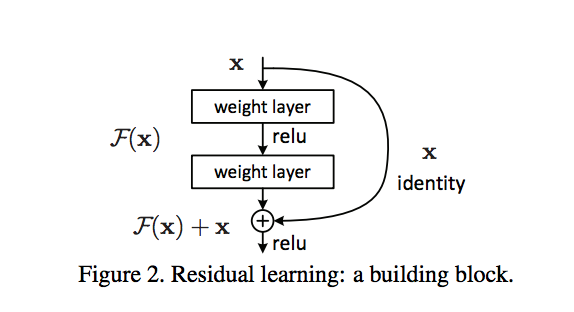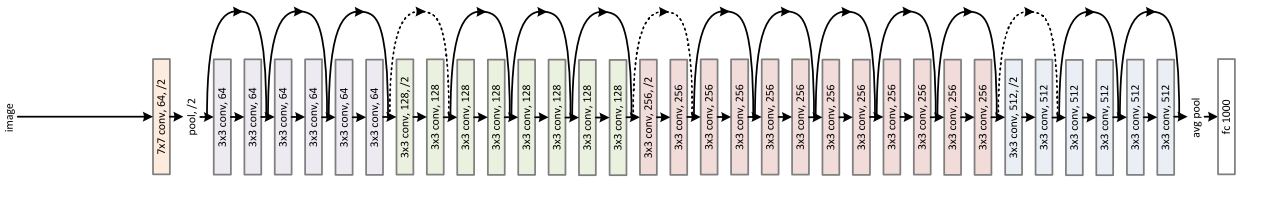# Skip connections and Residual blocks

Friday, September 7, 2018

Deep neural networks are difficult to train. They also have vanishing or exploding gradient problems. Batch normalization helps, but with the increase in depth, the network has trouble reaching convergence.

When deeper networks are able to start converging, a degradation problem has been exposed: with the network depth increasing, accuracy gets saturated (which might be unsurprising) and then degrades rapidly. Unexpectedly, such degradation is not caused by overfitting, and adding more layers to a suitably deep model leads to higher training error.

One solution to this problem was proposed by Kaiming He et. al in Deep Residual Learning for Image Recognition1 to use Resnet blocks, which connect the output of one layer with the input of an earlier layer. These skip connections are implemented as follows.Usually, a deep learning model learns the mapping, M, from an input x to an output y i.e.

$M(x) = y$

Instead of learning a direct mapping, the residual function uses the difference between a mapping applied to x and the original input, x i.e.

$F(x) = M(x) - x$

The skip layer connection is used i.e.

$M(x) = F(x) + x$ $\text{or}$ $y = F(x) + x$

It is easier to optimize this residual function F(x) compared to the original mapping M(x).

The Microsoft Research team won the ImageNet 2015 competition using these deep residual layers, which use skip connections. They used ResNet-152 convolutional neural network architecture, which consists of 152 layers.This page is open source. Improve its content!